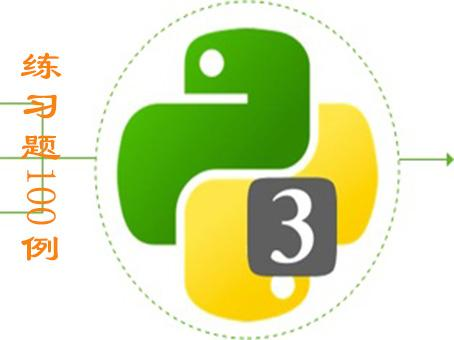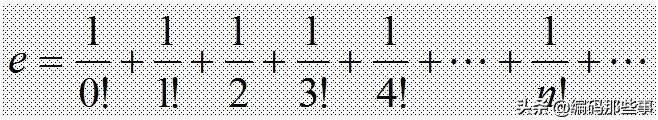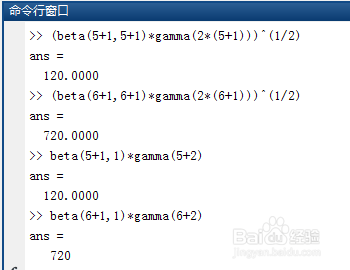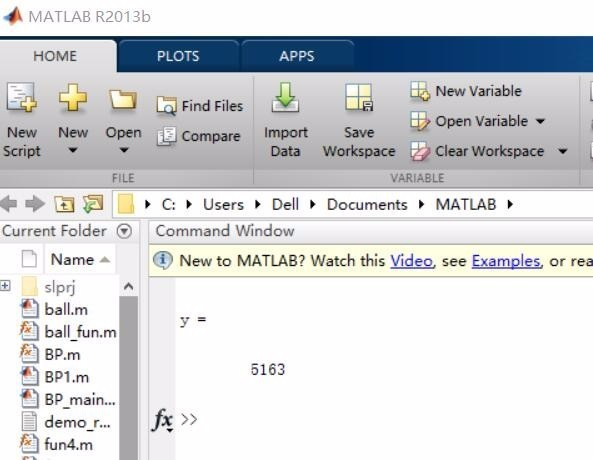• 计算n阶行列式
千次阅读
2020-03-16 13:45:38

# 摘要

本文主要介绍如何用拉普拉斯展开计算计算n阶行列式的值。

# 行列式

行列式（Determinant）是数学中的一个函数，将一个 n × n {n\times n} 的矩阵 A {A} 映射到一个纯量，记作 det ⁡ ( A ) 或 ∣ A ∣ {\det(A)}或{|A|} 。 ——摘自维基百科

说白了就是一个 n × n {n\times n} 的特殊矩阵。
但是虽然它跟矩阵很像，但也是两种不同的东西。

# 行列式的值

一个 2 × 2 {2\times 2} 的行列式，又被称为二阶行列式。
对于行列式：A= [ 1 2 3 4 ] {\begin{bmatrix}1&2\\3&4\end{bmatrix}}
其值为：A = 1 × 4 − 2 × 3 = − 2 {1\times 4 - 2\times 3 = -2}
3 × 3 {3\times 3} 的行列式，又被称为三阶行列式。
对于行列：B= [ 1 2 3 4 5 6 7 8 9 ] {\begin{bmatrix}1&2&3\\4&5&6\\7&8&9\end{bmatrix}}
其值为：
∣ B ∣ = 1 ⋅ ∣ 5 6 8 9 ∣ − 2 ⋅ ∣ 4 6 7 9 ∣ + 3 ⋅ ∣ 4 5 7 8 ∣ |B|=1\cdot {\begin{vmatrix}5&6\\8&9\end{vmatrix}}-2\cdot {\begin{vmatrix}4&6\\7&9\end{vmatrix}}+3\cdot {\begin{vmatrix}4&5\\7&8\end{vmatrix}}
= 1 ⋅ ( − 3 ) − 2 ⋅ ( − 6 ) + 3 ⋅ ( − 3 ) = 0 {\displaystyle {}=1\cdot (-3)-2\cdot (-6)+3\cdot (-3)=0}

以上对于三阶行列式的计算方式是：拉普拉斯展开。

## 拉普拉斯展开

在数学中，拉普拉斯展开（或称拉普拉斯公式）是一个关于行列式的展开式。将一个 n × n n×n 的行列式进行拉普拉斯展开，即是将其表示成关于行列式的某一行（或某一列）的n个元素与其代数余子式乘积的和。

## 余子式

对于行列式：B= [ 1 2 3 4 5 6 7 8 9 ] {\begin{bmatrix}1&2&3\\4&5&6\\7&8&9\end{bmatrix}}

[ 5 6 8 9 ] {\begin{bmatrix}5&6\\8&9\end{bmatrix}} 是其去掉第一行和第一列后的一个余子式。

[ 4 6 7 9 ] {\begin{bmatrix}4&6\\7&9\end{bmatrix}} 是其去掉第一行和第二列后的一个余子式。

在n阶行列式中，把所在的第i行与第j列划去后，所留下来的n-1阶行列式为余子式。

## 代数余子式

在n阶行列式中，把元素 a i j a_{ij} 所在的第 i i 行和第 j j 列划去后，留下来的n-1阶行列式叫做元素 a i j a_{ij} 的余子式，记作 M i j M_{ij} M i j × ( − 1 ) i + j M_{ij}\times(-1)^{i+j} 记为 A i j A_{ij} A i j A_{ij} 叫做元素 a i j a_{ij} 的代数余子式

对于行列式：B = [ 1 2 3 4 5 6 7 8 9 ] {\begin{bmatrix}1&2&3\\4&5&6\\7&8&9\end{bmatrix}}

a 11 的 代 数 余 子 式 为 ： − 1 ( 1 + 1 ) × ∣ 5 6 8 9 ∣ a_{11} 的代数余子式为：-1^{(1+1)}\times{\begin{vmatrix}5&6\\8&9\end{vmatrix}}

a 12 的 代 数 余 子 式 为 ： − 1 ( 1 + 2 ) × ∣ 4 6 7 9 ∣ a_{12} 的代数余子式为：-1^{(1+2)}\times{\begin{vmatrix}4&6\\7&9\end{vmatrix}}

a 13 的 代 数 余 子 式 为 ： − 1 ( 1 + 3 ) × ∣ 4 6 7 9 ∣ a_{13} 的代数余子式为：-1^{(1+3)}\times{\begin{vmatrix}4&6\\7&9\end{vmatrix}}

根据拉普拉斯展开，按第一行将行列式展开就得到：
∣ B ∣ = a 11 × A 11 + a 12 × A 12 + a 13 × A 13 |B| = a_{11} \times A_{11} + a_{12}\times A_{12} + a_{13}\times A_{13}

= 1 ⋅ ∣ 5 6 8 9 ∣ − 2 ⋅ ∣ 4 6 7 9 ∣ + 3 ⋅ ∣ 4 5 7 8 ∣ = 1\cdot {\begin{vmatrix}5&6\\8&9\end{vmatrix}}-2\cdot {\begin{vmatrix}4&6\\7&9\end{vmatrix}}+3\cdot {\begin{vmatrix}4&5\\7&8\end{vmatrix}}

= 1 ⋅ ( − 3 ) − 2 ⋅ ( − 6 ) + 3 ⋅ ( − 3 ) = 0 {=1\cdot (-3)-2\cdot (-6)+3\cdot (-3)=0}

# 代码实现:

计算n阶行列式的值的代码实现就是模拟拉普拉斯展开,假定以第一行展开， 不断的n阶行列式降阶， 直到n等于2时可以直接交叉计算，所以我们可以用递归的思路写。
比如一个5阶行列式，按第一行展开，将其转化为5个4阶行列式，然后将这5个4阶行列式转化为5*4个3阶，最后转化为2阶。

先给出一道模板题：
试题 算法提高 计算行列式

代码：

	import java.io.*;

public class Main{
static BufferedWriter out = new BufferedWriter(new OutputStreamWriter(System.out));

public static int Int(String s){return Integer.parseInt(s);}

public static void copy(int[][]A, int[][] A1, int i, int len) throws IOException{
for(int x = 1; x < len; x++)
for(int y = 0, j = 0; j < len; j++)
if(j != i) {
A1[x-1][y++] = A[x][j];
}
}

public static int F(int[][] A, int len)throws Exception{
int res = 0;
if(len == 1)return A;
if(len == 2){
return A*A - A*A; // 递归出口
}
else{
int A1[][] = new int;
for(int i = 0; i < len; i++){
copy(A, A1, i, len);// 得到余子式
res += Math.pow(-1, i) * A[i] * F(A1, len-1); //递归式
}
}
return res;
}

public static void main(String[] args) throws Exception{
int n;

int arr[][] = new int;

for(int i = 0; i < n; i++){
for(int j = 0; j < n; j++){
arr[i][j] = Int(s[j]);
}
}

out.write(F(arr, n) + "\n");
out.flush();
}
}


以上代码中用了一个递归函数求解行列式的值，用一个copy函数求解余子式的值，代码很简单，不再多解释了。算法 java 行列式
更多相关内容
• n的阶乘流程图(计算机n的阶乘流程图)2020-05-24 15:32:32共10个回答第一步:让s=1,i=1第二步输入要算的阶乘的对应的正整数n第三步让s=s*i,i=i+1第四步判断i是否大于n,若大于打印出S否则返回第三步s=1i=1inputnwhilei...

n的阶乘流程图(计算机n的阶乘流程图)

2020-05-24 15:32:32

共10个回答

第一步:让s=1,i=1第二步输入要算的阶乘的对应的正整数n第三步让s=s*i,i=i+1第四步判断i是否大于n,若大于打印出S否则返回第三步s=1i=1inputnwhilei用函数的递intf(intn){if(n>1)n=n*f(n-1);elseif(n==1)returnn;elseprintf(＂请输入正整数!＂);}代码大致就是这样,需要用递归.首先传入一个形参n,假如形参n>1那么执行递归,假如n=1则返回n的值.假如n<=0则提示输入正整数.我就在这简要写,自己整理一、1.开始2.乘积Mul初始化(1)3.循环变量i=14.Mul=Mul*i5.i=i+16.判断i是否大于n,大于继续,否则跳到步骤47.输出Mul8.结束1、输入一个数n2、赋值k=1,m=13、比较n是否大于k,若大于,跳至5;否则继续4、计算m=m*k,k=k+1,返回35、输出m摘要:本文提供了2个计算阶乘的程序.第1个程序采用在C中嵌入汇编代码的方法,改进上篇中了程序2的瓶颈部分,使速度提高到原先的3倍多.第2个程序进一步改进了classTest{staticintloop(intmax,intmin){intv,m,n;v=0;m=max;n=min;while(m-->n){v+=m;}returnv;};publicstaticvoidmain(String[]args){intrs=Test.loop(10,0);System.out.println(rs);}}可以用递归函数:#include#includelongdigui(intn);intmain(){intn;longdg;cout<>n;dg=digui(n);cout<最基础的思路,是逐个求阶乘,并累加.不过由于阶乘是从1乘到n,所以每个数都单独求一次阶乘,会有很多重复运算,影响效率.所以更快捷的方式是,在上一个数的阶乘基础上,直接乘上本身,得到当前数的阶乘.以此为主导,代码如下:#includeintmain(){intn,i,n1=1,s=0;scanf(＂%d＂,&n);//输入n值.for(i=1;i{n1*=i;//计算i的阶乘.s+=n1;//累加.}printf(＂%d\n＂,s);//输出结果.}importjava.util.Scanner;publicclassKnownTest{publicstaticvoidmain(String[]args){Scannersc=newScanner(System.in);System.out.println(＂输入一个数字＂);intn=sc.nextInt();System.out.println(＂结果是:＂+f(n));}publicstaticintf(intn){intsum=1;for(inti=1;isum*=i;}returnsum;}}展开全文• 1 math.factorial(x) 用python计算n的阶乘的方法。（含示例代码） 2. reduce函数 用python计算n的阶乘的方法。python里怎么分享n的阶乘就是那个n的阶层到底是多少的？非常简单的打开看一下这个辅导资料。输入n 计算n...

python中n的阶乘的算法？

1 math.factorial(x) 用python计算n的阶乘的方法。（含示例代码） 2. reduce函数 用python计算n的阶乘的方法。

python里怎么分享n的阶乘就是那个n的阶层到底是多少的？非常简单的打开看一下这个辅导资料。

输入n 计算n的阶乘，要用递归算法，python，大def fact(n):if n==0:return 1else:return n*fact(n-1)n=int(input())print(fact(n))习惯用一种固执的方式仰望着，仰望一片花海。那离幸福最近的地方。

利用python函数计算n的阶层

阶乘有一个经典的递归调用算法。以下是示例代码。 def jiecheng(n): if n==1 or n==0: return 1 return n*jiecheng(n-1)print('5的阶乘为%s'%jiecheng(5))你说，你喜欢雨。小编试着找出所有雨中的美好；尽管，小编不喜欢雨。

（急）用python计算阶乘如果你还在小编身边，也许小编就不会难过得痛苦万分。

小编需要用python列出一个阶乘的表。 小编不太清楚python的阶乘公式 以阶乘reduce为逐次操作list里的每项，接收的参数为 2个,最后返回的为一个结果 >>> def myadd(x,y): >>> return x*y >>> sum=reduce(myadd,(1,2,3,4,5)) >>> 120希望你能找到那个能抱着你睡觉的人，让你不再抱着娃娃和被子入睡!

补充结果 Python中 键盘输入一个正整数n并计算这个s=1n=int(input("请输入一个整数n:"))for i in range(______): s=______代码示例如下： 运行结果：当风吹起的时候，会想你。当风停止的时候，还是会想你。当路灯闪烁的时候，会想你。从那个路灯到家里步，每步都在想你。知道吧，不是因为伤心而落泪，是因为起风了。

用Python算n的阶乘，n的大小由用户输入。并且把计def factorial(n): if n == 1: return 1 else: return n * factorial(n - 1)input_num = input()try: with open('jc.txt', 'w') as file: file.write(str(factorial(int(input_num))))except IOError as e: print('error', e)许多时候，让小编们放不下的，其实并不是对方，而是那些逝去的共同回忆。

编程程序分享n的阶乘的计算实现方法，其中n值由用户输入

#include int jieCheng(int n){ if (1 == n || 0 == n) { return 1; } return n*jieCheng(n -1);}int main(){ printf("请输入n的值：\n"); int n = 0; scanf("%d", &n); printf("%d。 = %d \n", n, jieCheng(n)); return 0;}运行结果：真正成功的人生，不在于成就的大小，而在于你是否努力地去实现自小编，喊出自己的声音，走出属于自己的道路。

python 编写一个使阶乘计算器的程序 分享助小编的愿望是，可以选择性失忆，一觉醒来忘记你，彻彻底底的忘掉你。

用。表示阶乘，不重置的话，计算出来的是1。+1。2。+1。2。3。+1。2。3。4。+1。2。3。4。5。, 所以是34863。因为不重置，每次都在前一项的基础上乘了一个i。，但是小编们只需要在前一项的基础上乘以i就行了，所以多乘了。重置的话，虽然是正确的。

请编写一个程序实现分享n的阶乘（即n。），并打印输出结果。要分享包括两个函def little_than_50(x): if x 不要拿小编跟任何人比，小编最讨厌有人拿小编跟别人比，小编不是谁的影子也不是谁的替代品，更不是谁能退而分享其次的选择。

展开全文• 编写一个matlab文件，求copy1到n的阶2113乘之和。其代码编写的目5261的，就是学会自4102定义函数的编写，循环语句的使用1653。实现代码如下：function ex4()x=input('请输入正整数n：')s=0;for n=1:xy=exfun(n);s=s+...

编写一个matlab文件，求copy1到n的阶2113乘之和。其代码编写的目5261的，就是学会自4102定义函数的编写，循环语句的使用1653。实现代码如下：function ex4()x=input('请输入正整数n：')s=0;for  n=1:xy=exfun(n);s=s+y;endsendfunction y=exfun(x)y=1;for j=1:xy=y*j;endend运行效果更多追问追答追问我运行一下，可以后就采纳可以解释一下第八行s的意思吗？追答S是总和追问那为什么要把下一个for循环放到下面，这样的话程序执行一半不是应该就停了吗？大神，求解答追答下面的循环的作用是求n！追问多谢了，采纳晚了，抱歉，function a=fac(n)if n==0    a=1;else    a=0;    for i=1:n        b=1;        for j=1:i            b=b*j;        end        a=a+b;    endendwww.mh456.com防采集。

hs_fun.m function fx=hs_fun(x) if x>=100 exit end prd=1; for k=1:x prd=prd*k; end prd >> hs_fun(5) prd = 120

一、计数、求和、求阶乘等简单算法 例：用随机函数产生100个[0，99]首先确定x插在数组中的位置P；(可由以下语句实现) #define N 10 void一个c的 int f(int a) { if(a<=1) return a; else return a*f(a-1); }

用matlab如何编写一个函数文件,实现n!的方法。 如下参考： 1.首先在计算机上打开matlab软件，如下图所示。 2.点击打开页面“主页”下的“新建”按钮。 3.然后在打开的下拉菜单中选择“function”选项。 4.可以打开函数编辑器，在那里可以设置函数的输内容来自www.mh456.com请勿采集。

展开全文• 使用for循环计算n的阶乘 #include <stdio.h> int main() { int i = 0; int n = 0; int ret = 1; scanf("%d",&n); //例如输入5 for(i=1; i<=n;i++) { ret = ret * i; } printf(“ret = %d\n”,ret); ...c#
• 我们将编写一个程序，用Python计算以下系列。检查我们要编写的程序的示例输入和输出。Input:343+33+333+3333Output:3702Input:555+55+555+5555+55555Output:61725因此，我们将有两个数字，并且我们必须计算如上 生成...
• 设计一个shell程序计算n的阶乘，要求： （1）从命令行接收参数n； （2）最后输出计算的结果。 $cat factorial.sh #！/bin/bash echo “Please input number:” read n result=1 i=1 while[$i -le \$n] do result=`...linux shell
• ## c语言计算n的阶乘

千次阅读 多人点赞 2020-03-03 10:02:34
输入一个整数n，计算n的阶乘 1<=n<=1000 我们第一印象首先想到的就是用循环来直接计算n的阶乘，可是阶乘的时间复杂度是最高的，也就是说对于数字较大的阶乘我们无法通过直接计算来获得。 那么这么来计算较大...
• //递归方法计算一个数的阶乘 #include<stdio.h> #include<stdlib.h> int jiecheng(int f) { int n = 0; if (f < 0) printf("您的输入非法"); else if (0 == f || 1 == f) n = 1; else { n...递归思想
• C语言：利用函数递归实现计算n!。 #include<stdio.h> int fun(int n) { if(n==0||n==1) { n=1; } else { n=n*fun(n-1); } } int main() { int x,y; printf("请输入一个数字:\n"); scanf("%d",&...
• 这里介绍一下python中n次方运算的三种书写形式，代码如下： # -*- coding:utf-8 -*- """ author: 15025 time: 10.08.2021 18:12:20 software: PyCharm Description: """ import numpy as np class PythonStudy: ...python
• ## Python定义计算N的阶乘的函数

万次阅读 多人点赞 2019-06-29 21:28:57
定义计算N的阶乘的函数 1）使用循环计算阶乘 def frac(n): r = 1 if n<=1: if n==0 or n==1: return 1 else: print('n 不能小于0') else: for i in range(1, n+1): ...python
• 现有数列：1，2，3，4，……，n计算并输出其前n项的平方和，即求：‪‬‪‬‪‬‪‬‪‬‮‬‪‬‮‬‪‬‪‬‪‬‪‬‪‬‮‬‪‬‫‬‪‬‪‬‪‬‪‬‪‬‮‬‪‬‭‬‪‬‪‬‪‬‪‬‪‬‮‬‭‬‫‬‪‬‪‬...python 算法
• 例如：若输入的a为5，n为6，则要计算下面公式的值： 【输入形式】 从标准输入读入整数a和n，两者之间以一个空格分隔。 【输出形式】 在标准输出上输出公式的计算结果。 【样例1输入】 5 6 【样例1输出】 ...大数相减 数组
• ## C/C++ 计算N!

千次阅读 2018-09-28 09:46:56
编写程序，输入整数n（0 ≤ N ≤ 10000），计算n！ 输入 有多组数据，每组一个n 输出 每组占1行 样例输入 1 2 3 15 20 样例输出 1 2 6 1307674368000 2432902008176640000 #include &lt;stdio.h&...
• 利用for循环计算n!的值。 【分析】 n!=1×2×3×···×n 代码如下： 注：代码仅供参考，不只有一种解法。 #include<cstdio> int main(){ long long s=1; int n; scanf("%d",&n); for(int i=1;i<...c++
• mmn n C m n − = 要求：（a）编写一个函数计算 n! （b）编写主函数，由键盘输入 n 和 m，调用（a）中的函数完成计算。 （c）输入 n 和 m 要给出提示，并检查 n 和 m 的合理性，不合理的输入应输出错误信 息，并不...c语言
• 递归(recursion)算法 – 计算n！（n的阶乘） ①n！= n × (n-1) × (n-2) × …× 2 × 1 ② n！= 1 (n = 0 或 n = 1) n(n-1)！ (n > 1) 使用②进行递归运算 public static void main(String[] args) { ...java 递归 算法 阶乘
• #n! #定义n!的计算函数公式 def factorial(n): if n == 0: return 1 else: return n*factorial(n-1) #计算5！ jc=factorial(5) print(jc) n=0，return 1 n=1, return 1*factorial(0)=1*1 n=2, return 2*...
• int main(){ int n; printf("请输入n:"); scanf("%d",&amp;n); int i=1,mul=1; do { mul=mul*i; i++; }while(i&lt;=n); printf("%d\n",mul); return ...
• #include int func(int j) { ... int n;... printf("请输入一个整型数N：");  scantf("%d",&n);  int i;  for(i=0;i<n;i++)  {  printf("%d!=%d\n",i,func(i));  } }
• //计算n的阶乘 #define _CRT_SECURE_NO_WARNINGS #include <stdio.h> #include <stdlib.h> int main(){  int n, i, s = 1;  printf("请输入一个整数\n");  scanf("%d", &n); ...c语言
• 方法一：循环 public static void main(String[] args) { Scanner sc = new Scanner(System.in); System.out.println("输入一个正数：");... System.out.println("该数的阶乘是："+circulation(n)); } // ...java
• ## 2.计算n的阶层

千次阅读 2019-04-07 13:07:50
解析 n!公式有以下两种表达形式 第一种 第二种 步骤 Step1.当n=0时，计算0的阶层（0！...Step2....，反复调用求阶乘函数本身，直到n=0，则可正常计算出n的阶乘 ...//计算n的阶层 unsigned fac(unsign...C/C++
• double fact_s(int n); int main() { int i,n; printf("Input n:"); scanf("%d",&n); for(i=1;i<=n;i++) printf("%3d!=%.0f\n",i,fact_s(i)); return 0; } double fact_s(int n) { static double f=1...算法 c语言
• c语言计算n阶乘的方法：首先创建一个脚本文件，并编写头文件；然后定义一个“i”变量用来循环，以及定义变量“n”；接着用“sum”来保存结果；最后运行该脚本文件即可实现n阶乘。C语言中求阶乘的计算其实就是从1到n...
• 例题：请编写一个函数fun，它的功能是：计算n门课程的平均分，计算结果作为函数值返回。 例如，若有5门课程的成绩是88、92、80、61.5、55；则函数的值为75.30。 请勿改动主函数main与其它函数中的任何内容，仅在函数...
• 实验十 计算N!的实验 更多微机实验：https://blog.csdn.net/yxp189/column/info/39992 汇编语言-微机原理与接口技术-实验 注意：未经允许，请勿转载！ 转载请标明出处~ 适用课程：微机原理与接口技术（实验...汇编语言
• 分别用for、while和do-while循环语句以及递归方法计算n！，并输出算式 代码段 ​package package名; import java.util.Scanner; //引入包 public class A2_19 { static int factorial(int n) { //递归...Java 阶乘...